In geospatial applications we need to store and index different places or locations. Locations are represented with latitude and longitude.

Geospatial applications are most commonly the applications that have at least one feature dependent on geospatial data, for example: Google Maps, Searching nearby places in Yelp, looking for nearby drivers in Uber among many others. For users to query the massive databases of these apps, the indexing needs to be read efficient, since while searching for the nearby places users expect to see the results in real-time.

Spatial indexing is an increasingly important area of geo-spatial application design. The use-cases abound around us. Ride-sharing apps (Uber) need to be able to track the location of cars in near real-time, and provide user updates via extremely fast geo-queries. Facebook wants to let you know when your friends are nearby. The entities (Uber rides, Facebook users) and associated meta-data are stored in traditional tables and a spatial index is built separately with the coordinates of the entities. The spatial index allows special geometric queries to be executed efficiently.

With this index, these apps can efficiently query a database based on the spatial relationship of its entities. Some important queries that can be run from spatial indexes are listed below:
• Nearest neighbour (NN) queries: These queries are important for apps that provide nearby points of interests. Having a ‘nearby friends’ feature seems to be mainstream in social network applications, which can be easily powered by NN queries.

• Geo-distance Range Queries: These queries help retrieve entities within a specified range. Some use-cases include finding all cabs within a 5 miles radius from a user’s location.

A properly designed spatial indexing scheme is a central part of building high performance geo-apps.

#### Why is SQL Database not a very good choice for storing and querying geospatial data ?

Traditional DBMSs and their standard index implementations are able to index data based on various natural orderings. An important ordering that occurs in geospatial applications is the proximity ordering in a 2-D space. We could store location data in a SQL database. Each place will be stored in a separate row, uniquely identified by LocationID. Each place will have its longitude and latitude stored separately in two different columns, and to perform a fast search we should have indexes on both these fields. When there are millions of location data stored in a SQL DB, execution of any geospatial query, like finding locations within 2 miles of a given location, becomes very slow. SQL databases are not the best choice for storing and querying geospatial data.

One of the most efficient data structures for storing geospatial data is Quaftree. A quadtree is a tree data structure in which each node has zero or four children. It recursively divides a flat 2-D space into four quadrants.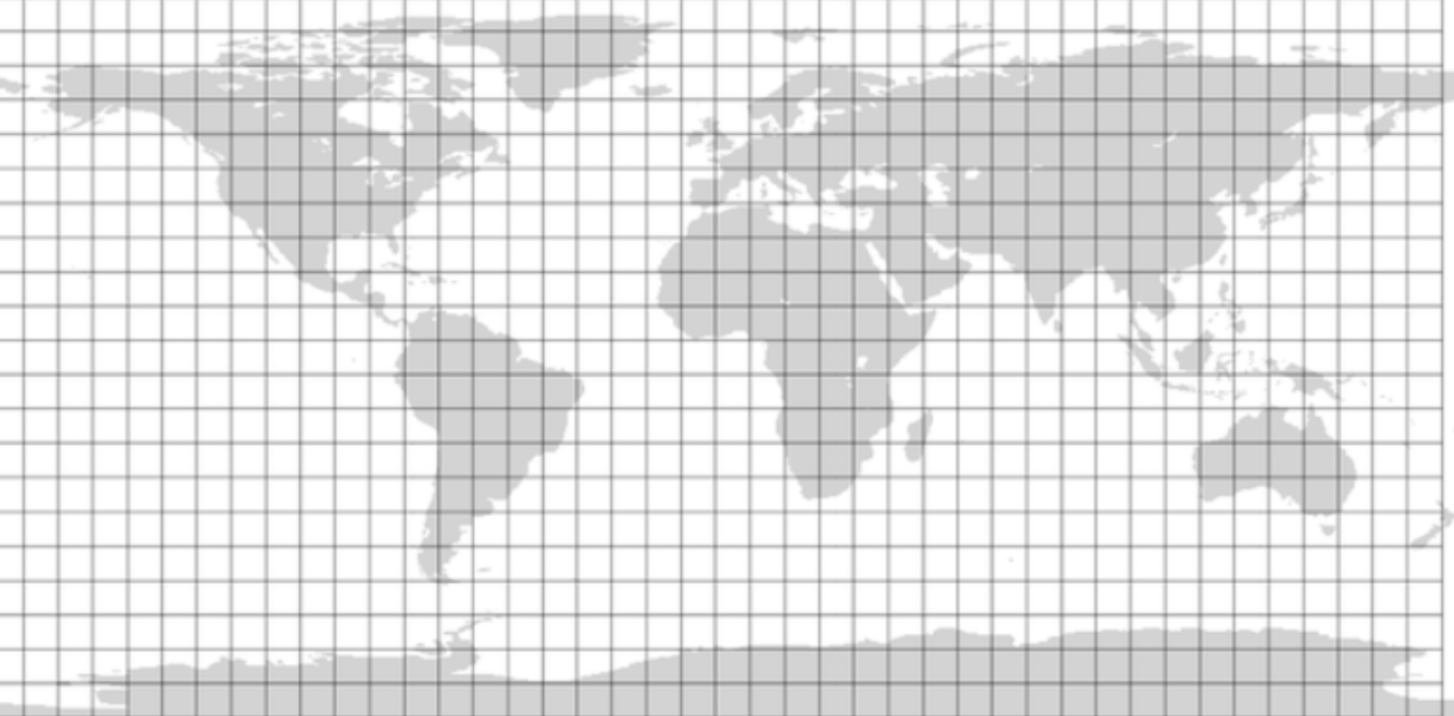#### We got a problem:

There is one problem here: the locations/places may not be uniformly distributed among grids in the quadtree. We can have a thickly dense area with a lot of places, and on the other hand, we can have areas which are sparsely populated.

This problem can be solved if we can dynamically adjust our grid size such that whenever we have a grid with a lot of places we break it down to create smaller grids. Let’s assume we don’t want to have more than 100 places in a grid so that we can have a faster searching. So, whenever a grid reaches this limit, we break it down into four grids of equal size and distribute places among them. This means thickly populated areas like New York City will have a lot of grids, and sparsely populated area like the Atlantic Ocean will have large grids with places only around the coastal lines.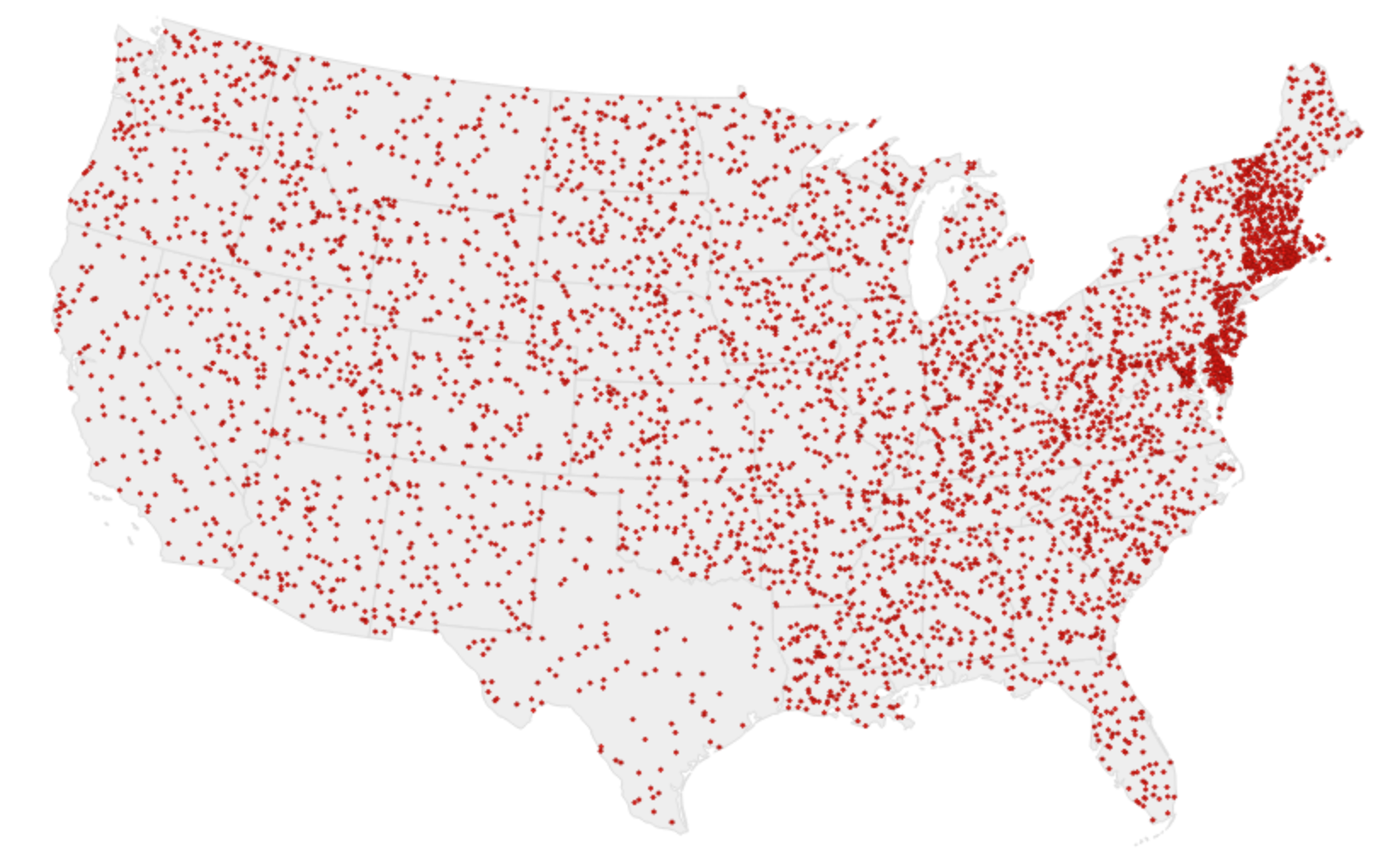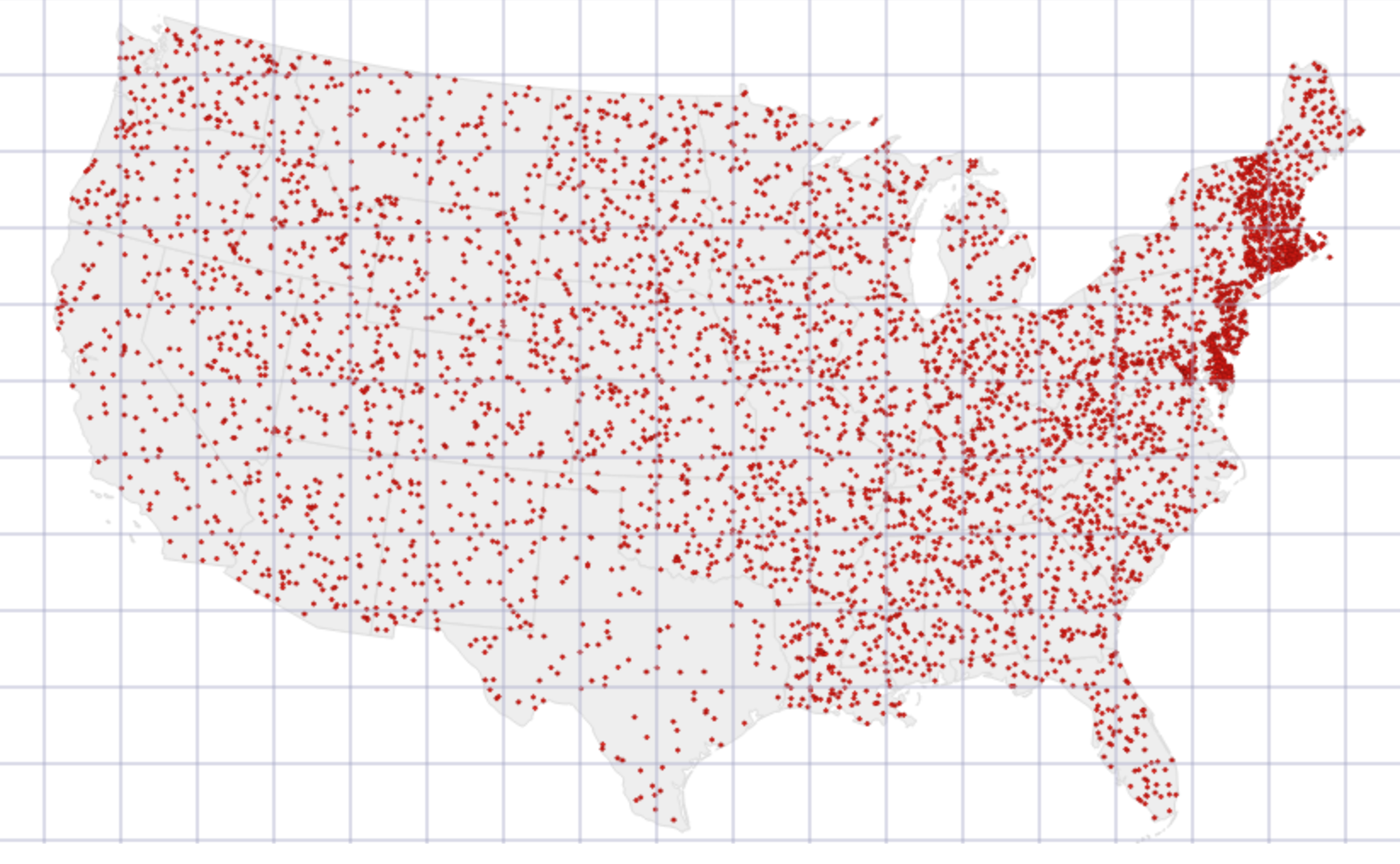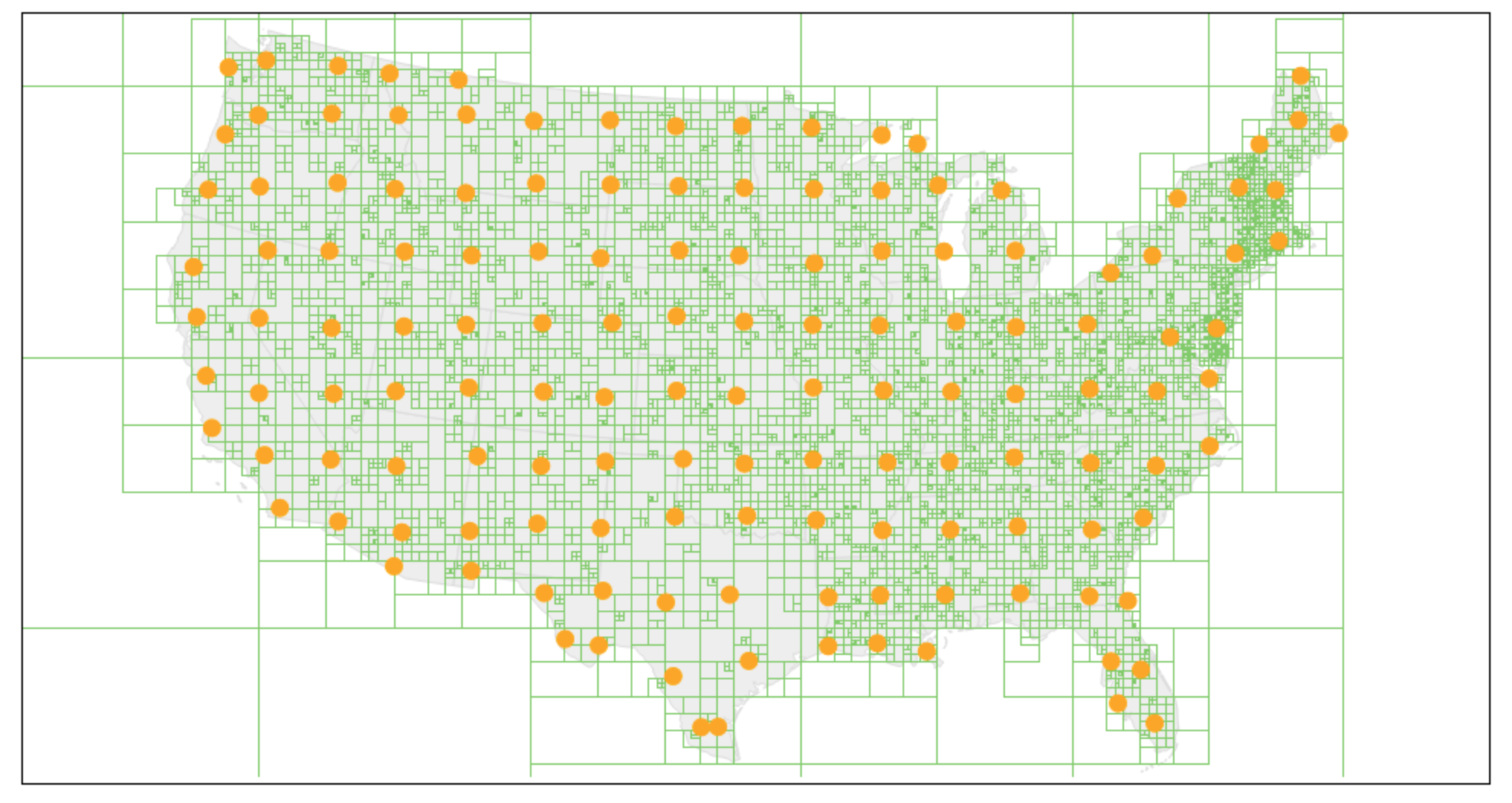Each node of Quadtree will represent a grid and will contain information about all the places in that grid. If a node reaches our limit of 100 places, we will break it down to create four child nodes under it and distribute places among them. In this way, all the leaf nodes will represent the grids that cannot be further broken down. So leaf nodes will keep a list of places with them.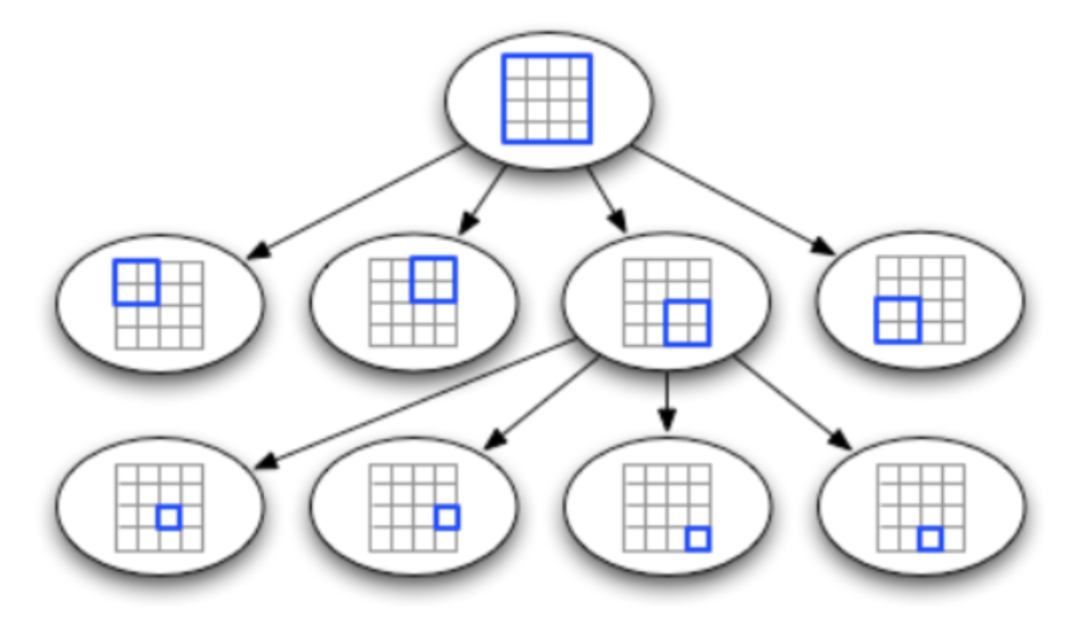#### Inserting data into a Quadtree:

Inserting data into a quadtree involves recursively determining which of the four children (quadrants) the data-point should occupy, until you reach a leaf-node (quadrant). If the elements in the leaf-nodes exceeds a pre-specified bucket size, the leaf node is split into four equal quadrants and the points are rearranged into their correct quadrants.

``````
insertInTree(root, data):
if not root
createLeafAndInsertNode(root, data)
else if root.isLeaf() and root.size() < BUCKET_SIZE:
else if root.isLeaf(): # Leaf node must be full
root.decomposeLeafNodeAndInsert(data)
# Find the appropriate sub-tree to insert node
else if root.northwest.isValidParent(data)
insertInTree(root.northwest, data)
else if root.southwest.isValidParent(data)
insertInTree(root.southwest, data)
else if root.southeast.isValidParent(data)
insertInTree(root.southeast, data)
else
insertInTree(root.northeast, data)
```
```

In a reasonably balanced quadtree, we would have insert implemented in logarithmic time. Worst case scenario is generally O(n) time, when the tree is extremely unbalanced.

#### Range Query:

A range query is an important function you could do with a Quadtree. It is generally of the form: retrieve all points within a spatial range. In real life applications, A ride-sharing service wants to expose an API to provide the cars available within 2 miles of a user’s location, or Facebook’s “nearby friends” feature wants to expose a list of friends in a user’s town.

``````
"""Pseudocode"""
getPointsInRange(root, range):
points = []
# If there is no intersection with the area, return
if not root.intersect(range):
return points
# Return all data points on a leaf node
if root.isLeaf():
points.append(root.getNodes())
return points
# Recursively append the points from the 4 quadrants
points.append(getPointsInRange(root.northwest, range))
points.append(getPointsInRange(root.northeast, range))
points.append(getPointsInRange(root.southeast, range))
points.append(getPointsInRange(root.southwest, range))
return points
```
```

#### Important Quadtree Spatial Indexing Implementation Notes:

The earth is not a flat 2-D surface, but it is an abstraction we make to help us divide the earth in a form that makes for easy representation. A proper quadtree implementation requires an optimal approach to representing the near spherical earth to a flat surface. GIS and other specialists generally make use of projections (like, Sinusoidal projection) for these representations.

Bucket Size makes a whole lot of difference:
Getting optimal performance out of your Quadtrees involves choosing the right tile-size (quadrant dimensions) depending on your particular use case. In some use-case, having the 2-D surface pre-divided into its composing grids may provide better performance at the expense of memory.

The Bucket size, the maximum number of data points in each leaf node, is also another variable that can be tuned to get better performance from the Quadtree. The ideal bucket-size depends on the use-case of the Quadtree. A quadtree with a bucket-size of 1 is quite easy to implement, but in real-world applications you get to have multiple entities in the same spatial region. The data points in the leaf node could be implemented with a LinkedList.

Image Processing and Image Compression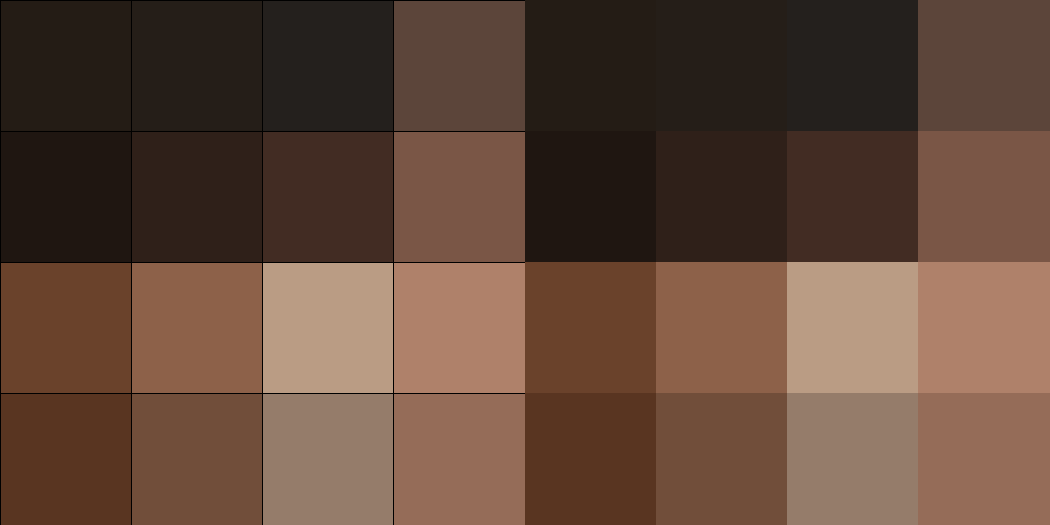#### Java Implementation:

``````
public class Interval2D<Key extends Comparable<Key>> {
public final Interval<Key> intervalX;   // x-interval
public final Interval<Key> intervalY;   // y-interval

public Interval2D(Interval<Key> intervalX, Interval<Key> intervalY) {
this.intervalX = intervalX;
this.intervalY = intervalY;
}

// does this 2D interval a intersect b?
public boolean intersects(Interval2D<Key> b) {
if (intervalX.intersects(b.intervalX)) return true;
if (intervalY.intersects(b.intervalY)) return true;
return false;
}

// does this 2D interval contain (x, y)?
public boolean contains(Key x, Key y) {
return intervalX.contains(x) && intervalY.contains(y);
}

// return string representation
public String toString() {
return intervalX + " x " + intervalY;
}
}

public class QuadTree<Key extends Comparable<Key>, Value>  {
private Node root;

// helper node data type
private class Node {
Key x, y;              // x- and y- coordinates
Node NW, NE, SE, SW;   // four subtrees: NorthWest , NorthEast, SouthEast, SouthWest
Value value;           // associated data

Node(Key x, Key y, Value value) {
this.x = x;
this.y = y;
this.value = value;
}
}

/***********************************************************************
*  Insert (x, y) into appropriate quadrant
***************************************************************************/
public void insert(Key x, Key y, Value value) {
root = insert(root, x, y, value);
}

private Node insert(Node h, Key x, Key y, Value value) {
if (h == null) return new Node(x, y, value);
//// if (eq(x, h.x) && eq(y, h.y)) h.value = value;  // duplicate
else if ( less(x, h.x) &&  less(y, h.y)) h.SW = insert(h.SW, x, y, value);
else if ( less(x, h.x) && !less(y, h.y)) h.NW = insert(h.NW, x, y, value);
else if (!less(x, h.x) &&  less(y, h.y)) h.SE = insert(h.SE, x, y, value);
else if (!less(x, h.x) && !less(y, h.y)) h.NE = insert(h.NE, x, y, value);
return h;
}

/***********************************************************************
*  Range search.
***************************************************************************/

public void query2D(Interval2D rect) {
query2D(root, rect);
}

private void query2D(Node h, Interval2D rect) {
if (h == null) return;
Key xmin = rect.intervalX.min();
Key ymin = rect.intervalY.min();
Key xmax = rect.intervalX.max();
Key ymax = rect.intervalY.max();
if (rect.contains(h.x, h.y))
StdOut.println("    (" + h.x + ", " + h.y + ") " + h.value);
if ( less(xmin, h.x) &&  less(ymin, h.y)) query2D(h.SW, rect);
if ( less(xmin, h.x) && !less(ymax, h.y)) query2D(h.NW, rect);
if (!less(xmax, h.x) &&  less(ymin, h.y)) query2D(h.SE, rect);
if (!less(xmax, h.x) && !less(ymax, h.y)) query2D(h.NE, rect);
}

/***************************************************************************
*  helper comparison functions
***************************************************************************/

private boolean less(Key k1, Key k2) { return k1.compareTo(k2) <  0; }
private boolean eq  (Key k1, Key k2) { return k1.compareTo(k2) == 0; }

/***************************************************************************
*  test client
***************************************************************************/
public static void main(String[] args) {
int M = Integer.parseInt(args);   // queries
int N = Integer.parseInt(args);   // points

// insert N random points in the unit square
for (int i = 0; i < N; i++) {
Integer x = (int) (100 * Math.random());
Integer y = (int) (100 * Math.random());
// StdOut.println("(" + x + ", " + y + ")");
st.insert(x, y, "P" + i);
}
StdOut.println("Done preprocessing " + N + " points");

// do some range searches
for (int i = 0; i < M; i++) {
Integer xmin = (int) (100 * Math.random());
Integer ymin = (int) (100 * Math.random());
Integer xmax = xmin + (int) (10 * Math.random());
Integer ymax = ymin + (int) (20 * Math.random());
Interval<Integer> intX = new Interval<>(xmin, xmax);
Interval<Integer> intY = new Interval<>(ymin, ymax);
Interval2D<Integer> rect = new Interval2D<>(intX, intY);
StdOut.println(rect + " : ");
st.query2D(rect);
}
}

}
```
```

#### Python Implementation:

``````
"""
# The below implementation stores and quickly retrieves items from a 2x2 rectangular grid area,
and grows in depth and detail as more items are added.
## Platforms
Python 2 and 3.
## Example Usage
from <> import Index
Setup the spatial index, giving it a bounding box area to keep track of.
The bounding box being in a four-tuple: (xmin, ymin, xmax, ymax).
spindex = Index(bbox=(0, 0, 100, 100))
Populate the index with items that you want to be retrieved at a later point,
along with each item's geographic bbox.
# this example assumes you have a list of items with bbox attribute
for item in items:
spindex.insert(item, item.bbox)
Then when you have a region of interest and you wish to retrieve items from that region,
just use the index's intersect method. This quickly gives you a list of the stored items
whose bboxes intersects your region of interests.
overlapbbox = (51, 51, 86, 86)
matches = spindex.intersect(overlapbbox)
"""

__version__ = "1.0.0"

#PYTHON VERSION CHECK
import sys
PYTHON3 = int(sys.version) == 3
if PYTHON3:
xrange = range

def _normalize_rect(rect):
if len(rect) == 2:
x1, y1 = rect
x2, y2 = rect
else:
x1, y1, x2, y2 = rect
if x1 > x2:
x1, x2 = x2, x1
if y1 > y2:
y1, y2 = y2, y1
return (x1, y1, x2, y2)

def _loopallchildren(parent):
for child in parent.children:
if child.children:
for subchild in _loopallchildren(child):
yield subchild
yield child

def __init__(self, item, rect):
self.item = item
self.rect = rect

def __eq__(self, other):
return self.item == other.item and self.rect == other.rect

def __hash__(self):
return hash(self.item)

"""
Internal backend version of the index.
The index being used behind the scenes. Has all the same methods as the user
index, but requires more technical arguments when initiating it than the
user-friendly version.
"""

def __init__(self, x, y, width, height, max_items, max_depth, _depth=0):
self.nodes = []
self.children = []
self.center = (x, y)
self.width, self.height = width, height
self.max_items = max_items
self.max_depth = max_depth
self._depth = _depth

def __iter__(self):
for child in _loopallchildren(self):
yield child

def _insert(self, item, bbox):
rect = _normalize_rect(bbox)
if len(self.children) == 0:
self.nodes.append(node)

if len(self.nodes) > self.max_items and self._depth < self.max_depth:
self._split()
else:
self._insert_into_children(item, rect)

def _remove(self, item, bbox):
rect = _normalize_rect(bbox)
if len(self.children) == 0:
self.nodes.remove(node)
else:
self._remove_from_children(item, rect)

def _intersect(self, rect, results=None, uniq=None):
if results is None:
rect = _normalize_rect(rect)
results = []
uniq = set()
# search children
if self.children:
if rect <= self.center:
if rect <= self.center:
self.children._intersect(rect, results, uniq)
if rect >= self.center:
self.children._intersect(rect, results, uniq)
if rect >= self.center:
if rect <= self.center:
self.children._intersect(rect, results, uniq)
if rect >= self.center:
self.children._intersect(rect, results, uniq)
# search node at this level
for node in self.nodes:
_id = id(node.item)
if (_id not in uniq and
node.rect >= rect and node.rect <= rect and
node.rect >= rect and node.rect <= rect):
results.append(node.item)
return results

def _insert_into_children(self, item, rect):
# if rect spans center then insert here
if (rect <= self.center and rect >= self.center and
rect <= self.center and rect >= self.center):
self.nodes.append(node)
else:
# try to insert into children
if rect <= self.center:
if rect <= self.center:
self.children._insert(item, rect)
if rect >= self.center:
self.children._insert(item, rect)
if rect > self.center:
if rect <= self.center:
self.children._insert(item, rect)
if rect >= self.center:
self.children._insert(item, rect)

def _remove_from_children(self, item, rect):
# if rect spans center then insert here
if (rect <= self.center and rect >= self.center and
rect <= self.center and rect >= self.center):
self.nodes.remove(node)
else:
# try to remove from children
if rect <= self.center:
if rect <= self.center:
self.children._remove(item, rect)
if rect >= self.center:
self.children._remove(item, rect)
if rect > self.center:
if rect <= self.center:
self.children._remove(item, rect)
if rect >= self.center:
self.children._remove(item, rect)

def _split(self):
quartwidth = self.width / 4.0
quartheight = self.height / 4.0
halfwidth = self.width / 2.0
halfheight = self.height / 2.0
x1 = self.center - quartwidth
x2 = self.center + quartwidth
y1 = self.center - quartheight
y2 = self.center + quartheight
new_depth = self._depth + 1
self.children = [_QuadTree(x1, y1, halfwidth, halfheight,
self.max_items, self.max_depth, new_depth),
self.max_items, self.max_depth, new_depth),
self.max_items, self.max_depth, new_depth),
self.max_items, self.max_depth, new_depth)]
nodes = self.nodes
self.nodes = []
for node in nodes:
self._insert_into_children(node.item, node.rect)

def __len__(self):
"""
Returns:
- A count of the total number of members/items/nodes inserted
into this quadtree and all of its child trees.
"""
size = 0
for child in self.children:
size += len(child)
size += len(self.nodes)
return size

MAX_ITEMS = 10
MAX_DEPTH = 20

"""
The top spatial index to be created by the user. Once created it can be
populated with geographically placed members that can later be tested for
intersection with a user inputted geographic bounding box. Note that the
index can be iterated through in a for-statement, which loops through all
all the quad instances and lets you access their properties.
Example usage:
>>> spindex = Index(bbox=(0, 0, 100, 100))
>>> spindex.insert('duck', (50, 30, 53, 60))
>>> spindex.insert('cookie', (10, 20, 15, 25))
>>> spindex.insert('python', (40, 50, 95, 90))
>>> results = spindex.intersect((51, 51, 86, 86))
>>> sorted(results)
['duck', 'python']
"""

def __init__(self, bbox=None, x=None, y=None, width=None, height=None, max_items=MAX_ITEMS, max_depth=MAX_DEPTH):
"""
Initiate by specifying either 1) a bbox to keep track of, or 2) with an xy centerpoint and a width and height.
Parameters:
- **bbox**: The coordinate system bounding box of the area that the quadtree should
keep track of, as a 4-length sequence (xmin,ymin,xmax,ymax)
- **x**:
The x center coordinate of the area that the quadtree should keep track of.
- **y**
The y center coordinate of the area that the quadtree should keep track of.
- **width**:
How far from the xcenter that the quadtree should look when keeping track.
- **height**:
How far from the ycenter that the quadtree should look when keeping track
- **max_items** (optional): The maximum number of items allowed per quad before splitting
up into four new subquads. Default is 10.
- **max_depth** (optional): The maximum levels of nested subquads, after which no more splitting
occurs and the bottommost quad nodes may grow indefinately. Default is 20.
"""
if bbox is not None:
x1, y1, x2, y2 = bbox
width, height = abs(x2-x1), abs(y2-y1)
midx, midy = x1+width/2.0, y1+height/2.0
super(Index, self).__init__(midx, midy, width, height, max_items, max_depth)

elif None not in (x, y, width, height):
super(Index, self).__init__(x, y, width, height, max_items, max_depth)

else:
raise Exception("Either the bbox argument must be set, or the x, y, width, and height arguments must be set")

def insert(self, item, bbox):
"""
Inserts an item into the quadtree along with its bounding box.
Parameters:
- **item**: The item to insert into the index, which will be returned by the intersection method
- **bbox**: The spatial bounding box tuple of the item, with four members (xmin,ymin,xmax,ymax)
"""
self._insert(item, bbox)

def remove(self, item, bbox):
"""
Removes an item from the quadtree.
Parameters:
- **item**: The item to remove from the index
- **bbox**: The spatial bounding box tuple of the item, with four members (xmin,ymin,xmax,ymax)
Both parameters need to exactly match the parameters provided to the insert method.
"""
self._remove(item, bbox)

def intersect(self, bbox):
"""
Intersects an input boundingbox rectangle with all of the items
Parameters:
- **bbox**: A spatial bounding box tuple with four members (xmin,ymin,xmax,ymax)
Returns:
- A list of inserted items whose bounding boxes intersect with the input bbox.
"""
return self._intersect(bbox)
```
```

#### Problem Solving:

It is really important that you got a clear understanding about the internals of Quadtree. To achieve that, we would solve a simple problem.

Problem Statement:
Given a n * n matrix grid of 0's and 1's only. We want to represent the grid with a Quad-Tree.

Return the root of the Quad-Tree representing the grid.

Notice that you can assign the value of a node to True or False when isLeaf is False, and both are accepted in the answer.

A Quad-Tree is a tree data structure in which each internal node has exactly four children. Besides, each node has two attributes:
• val: True if the node represents a grid of 1's or False if the node represents a grid of 0's.
• isLeaf: True if the node is leaf node on the tree or False if the node has the four children.

```class Node {
public boolean val;
public boolean isLeaf;
public Node topLeft;
public Node topRight;
public Node bottomLeft;
public Node bottomRight;
}
```

We can construct a Quad-Tree from a two-dimensional area using the following steps:
• If the current grid has the same value (i.e all 1's or all 0's) set isLeaf True and set val to the value of the grid and set the four children to Null and stop.
• If the current grid has different values, set isLeaf to False and set val to any value and divide the current grid into four sub-grids as shown in the photo.
• Recurse for each of the children with the proper sub-grid.The output represents the serialized format of a Quad-Tree using level order traversal, where null signifies a path terminator where no node exists below.

It is very similar to the serialization of the binary tree. The only difference is that the node is represented as a list [isLeaf, val].

If the value of isLeaf or val is True we represent it as 1 in the list [isLeaf, val] and if the value of isLeaf or val is False we represent it as 0.

Example 1:Input: grid = [[0,1],[1,0]]
Output: [[0,1],[1,0],[1,1],[1,1],[1,0]]
Explanation: The explanation of this example is shown below:
Notice that 0 represnts False and 1 represents True in the photo representing the Quad-Tree.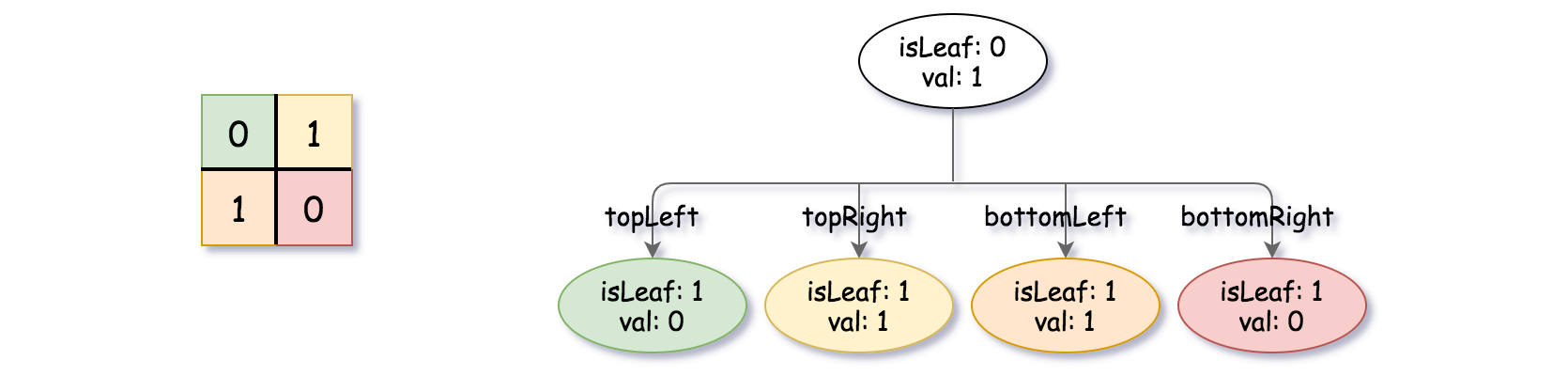Example 2: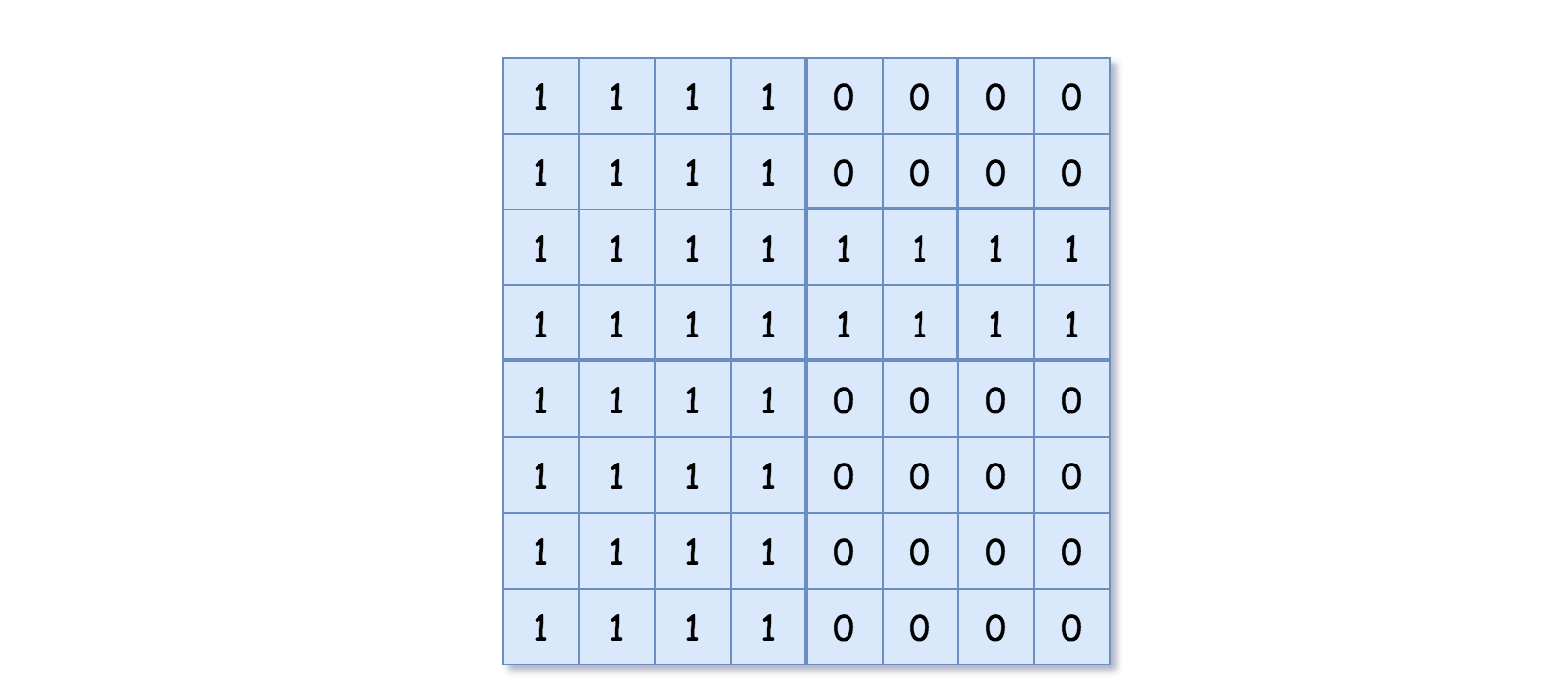Input: grid = [[1,1,1,1,0,0,0,0],[1,1,1,1,0,0,0,0],[1,1,1,1,1,1,1,1],[1,1,1,1,1,1,1,1],[1,1,1,1,0,0,0,0],[1,1,1,1,0,0,0,0],[1,1,1,1,0,0,0,0],[1,1,1,1,0,0,0,0]]

Output: [[0,1],[1,1],[0,1],[1,1],[1,0],null,null,null,null,[1,0],[1,0],[1,1],[1,1]]

Explanation: All values in the grid are not the same. We divide the grid into four sub-grids. The topLeft, bottomLeft and bottomRight each has the same value. The topRight have different values so we divide it into 4 sub-grids where each has the same value. Explanation is shown in the photo below: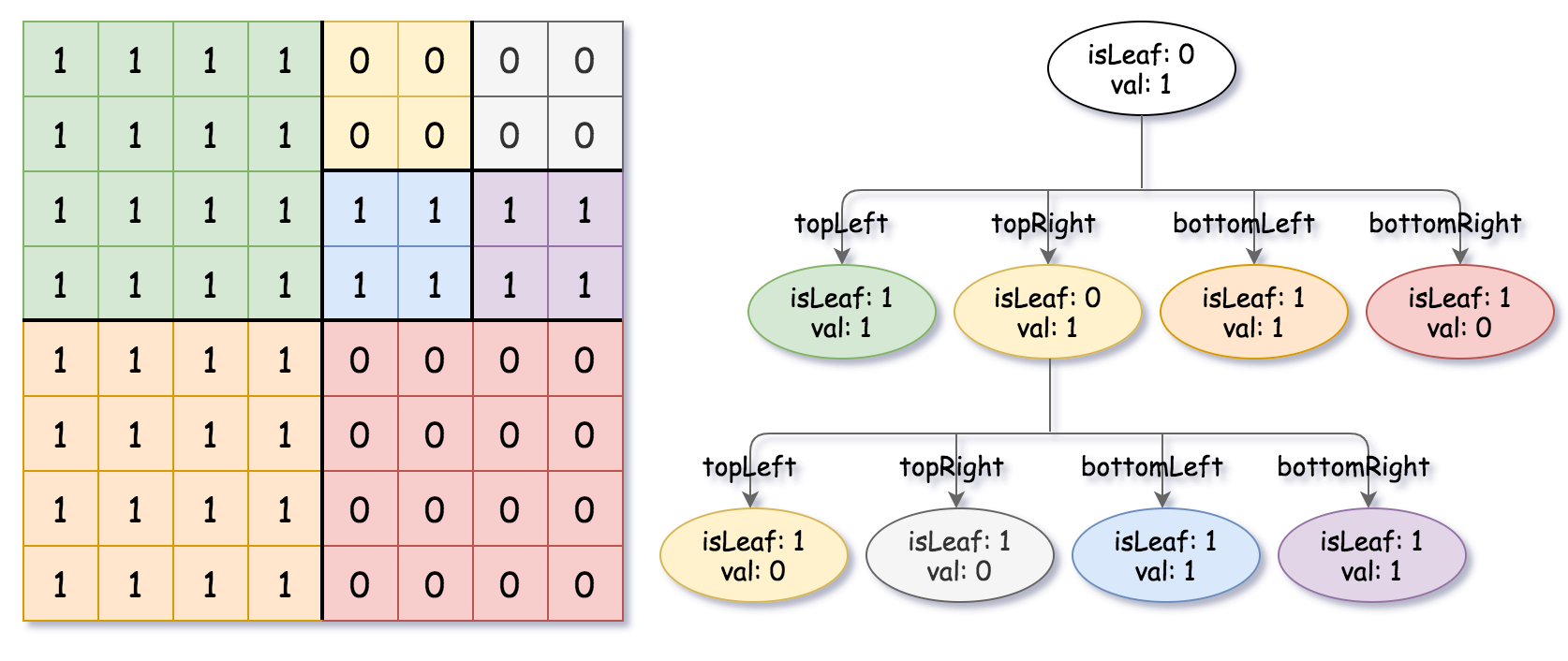Example 3:
Input: grid = [[1,1],[1,1]]
Output: [[1,1]]

Example 4:
Input: grid = []
Output: [[1,0]]

Example 5:
Input: grid = [[1,1,0,0],[1,1,0,0],[0,0,1,1],[0,0,1,1]]
Output: [[0,1],[1,1],[1,0],[1,0],[1,1]]

#### Solution:

``````
/*
// Definition for a QuadTree node.
class Node {
public boolean val;
public boolean isLeaf;
public Node topLeft;
public Node topRight;
public Node bottomLeft;
public Node bottomRight;

public Node() {
this.val = false;
this.isLeaf = false;
this.topLeft = null;
this.topRight = null;
this.bottomLeft = null;
this.bottomRight = null;
}

public Node(boolean val, boolean isLeaf) {
this.val = val;
this.isLeaf = isLeaf;
this.topLeft = null;
this.topRight = null;
this.bottomLeft = null;
this.bottomRight = null;
}

public Node(boolean val, boolean isLeaf, Node topLeft, Node topRight, Node bottomLeft, Node bottomRight) {
this.val = val;
this.isLeaf = isLeaf;
this.topLeft = topLeft;
this.topRight = topRight;
this.bottomLeft = bottomLeft;
this.bottomRight = bottomRight;
}
};
*/

class Solution {

public Node construct(int[][] grid) {
if (grid == null || grid.length == 0) {
return null;
}
return constructHelper(grid, 0, 0, grid.length - 1, grid.length - 1);
}

private Node constructHelper(int[][] grid, int columnBeginIndex, int rowBeginIndex, int columnEndIndex, int rowEndIndex) {
if (columnBeginIndex == columnEndIndex || areAllCellValuesSame(grid, columnBeginIndex, rowBeginIndex, columnEndIndex, rowEndIndex)) {
return new Node(grid[rowBeginIndex][columnBeginIndex] == 1, true);
}
int colMid = (columnEndIndex + columnBeginIndex) / 2;
int rowMid = (rowEndIndex + rowBeginIndex) / 2;
return new Node(
false,
false,
constructHelper(grid, columnBeginIndex, rowBeginIndex, colMid, rowMid),
constructHelper(grid, 1 + colMid, rowBeginIndex, columnEndIndex, rowMid),
constructHelper(grid, columnBeginIndex, 1 + rowMid, colMid, rowEndIndex),
constructHelper(grid, 1 + colMid, 1 + rowMid, columnEndIndex, rowEndIndex) );
}

private boolean areAllCellValuesSame(int[][] grid, int columnBeginIndex, int rowBeginIndex, int columnEndIndex, int rowEndIndex) {
for (int row = rowBeginIndex; row <= rowEndIndex; row++) {
for (int col = columnBeginIndex; col <= columnEndIndex; col++) {
if (grid[row][col] != grid[rowBeginIndex][columnBeginIndex]) {
return false;
}
}
}
return true;
}
}

```
```

#### The above content is written by: